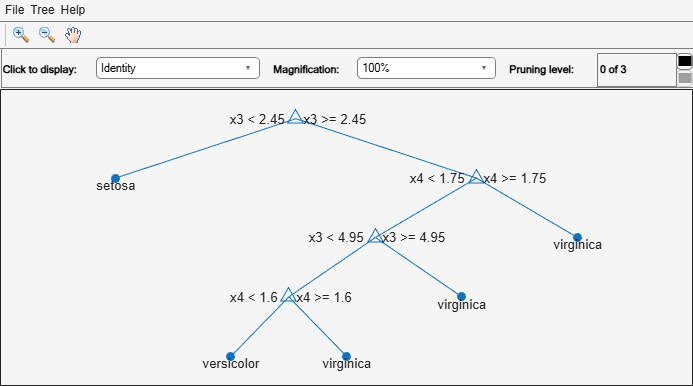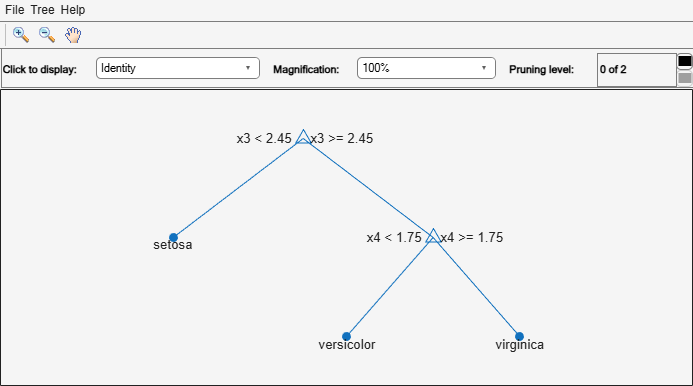Documentation

### This is machine translation

Mouseover text to see original. Click the button below to return to the English version of the page.

# resubLoss

Class: ClassificationTree

Classification error by resubstitution

## Syntax

```L = resubLoss(tree) L = resubLoss(tree,Name,Value) L = resubLoss(tree,'Subtrees',subtreevector) [L,se] = resubLoss(tree,'Subtrees',subtreevector) [L,se,NLeaf] = resubLoss(tree,'Subtrees',subtreevector) [L,se,NLeaf,bestlevel] = resubLoss(tree,'Subtrees',subtreevector) [L,...] = resubLoss(tree,'Subtrees',subtreevector,Name,Value) ```

## Description

`L = resubLoss(tree)` returns the resubstitution loss, meaning the loss computed for the data that `fitctree` used to create `tree`.

`L = resubLoss(tree,Name,Value)` returns the loss with additional options specified by one or more `Name,Value` pair arguments. You can specify several name-value pair arguments in any order as `Name1,Value1,…,NameN,ValueN`.

`L = resubLoss(tree,'Subtrees',subtreevector)` returns a vector of classification errors for the trees in the pruning sequence `subtreevector`.

```[L,se] = resubLoss(tree,'Subtrees',subtreevector)``` returns the vector of standard errors of the classification errors.

```[L,se,NLeaf] = resubLoss(tree,'Subtrees',subtreevector)``` returns the vector of numbers of leaf nodes in the trees of the pruning sequence.

```[L,se,NLeaf,bestlevel] = resubLoss(tree,'Subtrees',subtreevector)``` returns the best pruning level as defined in the `TreeSize` name-value pair. By default, `bestlevel` is the pruning level that gives loss within one standard deviation of minimal loss.

`[L,...] = resubLoss(tree,'Subtrees',subtreevector,Name,Value)` returns loss statistics with additional options specified by one or more `Name,Value` pair arguments. You can specify several name-value pair arguments in any order as `Name1,Value1,…,NameN,ValueN`.

## Input Arguments

expand all

 `tree` A classification tree constructed by `fitctree`.

### Name-Value Pair Arguments

Specify optional comma-separated pairs of `Name,Value` arguments. `Name` is the argument name and `Value` is the corresponding value. `Name` must appear inside quotes. You can specify several name and value pair arguments in any order as `Name1,Value1,...,NameN,ValueN`.

Loss function, specified as the comma-separated pair consisting of `'LossFun'` and a built-in, loss-function name or function handle.

• The following table lists the available loss functions. Specify one using its corresponding character vector or string scalar.

ValueDescription
`'binodeviance'`Binomial deviance
`'classiferror'`Classification error
`'exponential'`Exponential
`'hinge'`Hinge
`'logit'`Logistic
`'mincost'`Minimal expected misclassification cost (for classification scores that are posterior probabilities)
`'quadratic'`Quadratic

`'mincost'` is appropriate for classification scores that are posterior probabilities. Classification trees return posterior probabilities as classification scores by default (see `predict`).

• Specify your own function using function handle notation.

Suppose that `n` be the number of observations in `X` and `K` be the number of distinct classes (`numel(tree.ClassNames)`). Your function must have this signature

``lossvalue = lossfun(C,S,W,Cost)``
where:

• The output argument `lossvalue` is a scalar.

• You choose the function name (`lossfun`).

• `C` is an `n`-by-`K` logical matrix with rows indicating which class the corresponding observation belongs. The column order corresponds to the class order in `tree.ClassNames`.

Construct `C` by setting ```C(p,q) = 1``` if observation `p` is in class `q`, for each row. Set all other elements of row `p` to `0`.

• `S` is an `n`-by-`K` numeric matrix of classification scores. The column order corresponds to the class order in `tree.ClassNames`. `S` is a matrix of classification scores, similar to the output of `predict`.

• `W` is an `n`-by-1 numeric vector of observation weights. If you pass `W`, the software normalizes them to sum to `1`.

• `Cost` is a K-by-`K` numeric matrix of misclassification costs. For example, ```Cost = ones(K) - eye(K)``` specifies a cost of `0` for correct classification, and `1` for misclassification.

Specify your function using `'LossFun',@lossfun`.

For more details on loss functions, see Classification Loss.

Data Types: `char` | `string` | `function_handle`

`Name,Value` arguments associated with pruning subtrees:

Pruning level, specified as the comma-separated pair consisting of `'Subtrees'` and a vector of nonnegative integers in ascending order or `'all'`.

If you specify a vector, then all elements must be at least `0` and at most `max(tree.PruneList)`. `0` indicates the full, unpruned tree and `max(tree.PruneList)` indicates the completely pruned tree (i.e., just the root node).

If you specify `'all'`, then `resubLoss` operates on all subtrees (i.e., the entire pruning sequence). This specification is equivalent to using `0:max(tree.PruneList)`.

`resubLoss` prunes `tree` to each level indicated in `Subtrees`, and then estimates the corresponding output arguments. The size of `Subtrees` determines the size of some output arguments.

To invoke `Subtrees`, the properties `PruneList` and `PruneAlpha` of `tree` must be nonempty. In other words, grow `tree` by setting `'Prune','on'`, or by pruning `tree` using `prune`.

Example: `'Subtrees','all'`

Data Types: `single` | `double` | `char` | `string`

Tree size, specified as the comma-separated pair consisting of `'TreeSize'` and one of the following values:

• `'se'``loss` returns the highest pruning level with loss within one standard deviation of the minimum (`L`+`se`, where `L` and `se` relate to the smallest value in `Subtrees`).

• `'min'``loss` returns the element of `Subtrees` with smallest loss, usually the smallest element of `Subtrees`.

## Output Arguments

 `L` Classification loss, a vector the length of `Subtrees`. The meaning of the error depends on the values in `Weights` and `LossFun`. `se` Standard error of loss, a vector the length of `Subtrees`. `NLeaf` Number of leaves (terminal nodes) in the pruned subtrees, a vector the length of `Subtrees`. `bestlevel` A scalar whose value depends on `TreeSize`: `TreeSize` = `'se'` — `loss` returns the highest pruning level with loss within one standard deviation of the minimum (`L`+`se`, where `L` and `se` relate to the smallest value in `Subtrees`).`TreeSize` = `'min'` — `loss` returns the element of `Subtrees` with smallest loss, usually the smallest element of `Subtrees`.

## Examples

expand all

Compute the resubstitution classification error for the `ionosphere` data.

```load ionosphere tree = fitctree(X,Y); L = resubLoss(tree)```
```L = 0.0114 ```

Unpruned decision trees tend to overfit. One way to balance model complexity and out-of-sample performance is to prune a tree (or restrict its growth) so that in-sample and out-of-sample performance are satisfactory.

Load Fisher's iris data set. Partition the data into training (50%) and validation (50%) sets.

```load fisheriris n = size(meas,1); rng(1) % For reproducibility idxTrn = false(n,1); idxTrn(randsample(n,round(0.5*n))) = true; % Training set logical indices idxVal = idxTrn == false; % Validation set logical indices```

Grow a classification tree using the training set.

`Mdl = fitctree(meas(idxTrn,:),species(idxTrn));`

View the classification tree.

`view(Mdl,'Mode','graph');`The classification tree has four pruning levels. Level 0 is the full, unpruned tree (as displayed). Level 3 is just the root node (i.e., no splits).

Examine the training sample classification error for each subtree (or pruning level) excluding the highest level.

```m = max(Mdl.PruneList) - 1; trnLoss = resubLoss(Mdl,'SubTrees',0:m)```
```trnLoss = 3×1 0.0267 0.0533 0.3067 ```
• The full, unpruned tree misclassifies about 2.7% of the training observations.

• The tree pruned to level 1 misclassifies about 5.3% of the training observations.

• The tree pruned to level 2 (i.e., a stump) misclassifies about 30.6% of the training observations.

Examine the validation sample classification error at each level excluding the highest level.

`valLoss = loss(Mdl,meas(idxVal,:),species(idxVal),'SubTrees',0:m)`
```valLoss = 3×1 0.0369 0.0237 0.3067 ```
• The full, unpruned tree misclassifies about 3.7% of the validation observations.

• The tree pruned to level 1 misclassifies about 2.4% of the validation observations.

• The tree pruned to level 2 (i.e., a stump) misclassifies about 30.7% of the validation observations.

To balance model complexity and out-of-sample performance, consider pruning `Mdl` to level 1.

```pruneMdl = prune(Mdl,'Level',1); view(pruneMdl,'Mode','graph')```